Test: Steady State Time Domain Analysis of Type-A Chopper

# Test: Steady State Time Domain Analysis of Type-A Chopper

Test Description

## 5 Questions MCQ Test Power Electronics | Test: Steady State Time Domain Analysis of Type-A Chopper

Test: Steady State Time Domain Analysis of Type-A Chopper for Electrical Engineering (EE) 2023 is part of Power Electronics preparation. The Test: Steady State Time Domain Analysis of Type-A Chopper questions and answers have been prepared according to the Electrical Engineering (EE) exam syllabus.The Test: Steady State Time Domain Analysis of Type-A Chopper MCQs are made for Electrical Engineering (EE) 2023 Exam. Find important definitions, questions, notes, meanings, examples, exercises, MCQs and online tests for Test: Steady State Time Domain Analysis of Type-A Chopper below.
Solutions of Test: Steady State Time Domain Analysis of Type-A Chopper questions in English are available as part of our Power Electronics for Electrical Engineering (EE) & Test: Steady State Time Domain Analysis of Type-A Chopper solutions in Hindi for Power Electronics course. Download more important topics, notes, lectures and mock test series for Electrical Engineering (EE) Exam by signing up for free. Attempt Test: Steady State Time Domain Analysis of Type-A Chopper | 5 questions in 15 minutes | Mock test for Electrical Engineering (EE) preparation | Free important questions MCQ to study Power Electronics for Electrical Engineering (EE) Exam | Download free PDF with solutions
 1 Crore+ students have signed up on EduRev. Have you?
*Answer can only contain numeric values
Test: Steady State Time Domain Analysis of Type-A Chopper - Question 1

### In the dc-dc converter circuit shown, switch Q is switched at a frequency of 10 kHz with a duty ratio of 0.6. All components of the circuit are ideal, and the initial current in the inductor is zero. Energy stored in the inductor in mJ (rounded off to 2 decimal places) at the end of 10 complete switching cycles is ________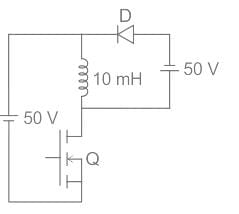Detailed Solution for Test: Steady State Time Domain Analysis of Type-A Chopper - Question 1

Identification of DC-DC Converter:

• Buck converter: Inductor is connected in series with the combination of the capacitor and load resistor.
• Boost converter: Inductor is connected in series with the supply voltage source.
• Buck-Boost converter: Inductor is connected in parallel to the series-connected switch and supply voltage source.

In a Buck-Boost converter, the inductor stores the energy for TON time and release the energy for the time TOFF
Given that, duty ratio (δ) = 0.6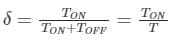⇒ TON = δT = 0.6 T
⇒ TOFF = (1 – δ) T = 0.4 T
So, the inductor stores the energy for a time of 0.6 T and releases the energy for 0.4 T.
So, for one cycle, the increase in inductor current is corresponding to a time of (0.6 T – 0.4 T) = 0.2 T
Frequency (f) = 10 kHz
The time period for one cycle,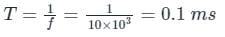The waveform of the inductor current is as shown below.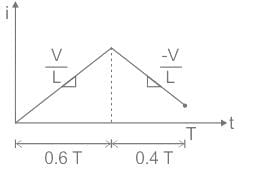From the waveform, rise in the inductor current for one cycle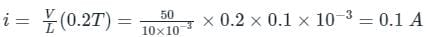Rise in the inductor current for 10 cycles = 0.1 × 10 = 1 A
Energy stored in the inductor for 10 cycles,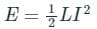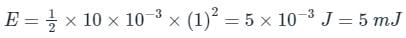*Answer can only contain numeric values
Test: Steady State Time Domain Analysis of Type-A Chopper - Question 2

### For the circuit shown in the figure, assume L to be large enough to ensure linear growth and decay of the current through it and have continuous current. The maximum value of the load current in amperes is _________ (Vs = 100 V, Vo = 50 V, L = 2mH, f = 20 kHz, R = 10 Ω)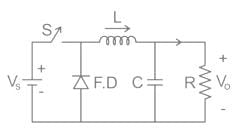Detailed Solution for Test: Steady State Time Domain Analysis of Type-A Chopper - Question 2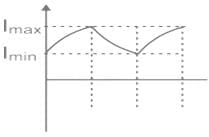The function of capacitor C across-load resistance R is to make the output voltage continuous.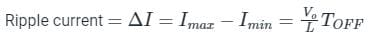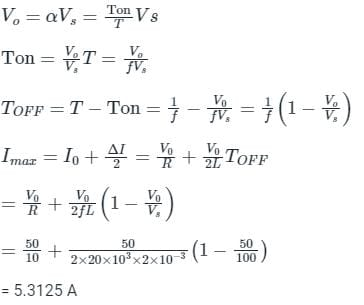Test: Steady State Time Domain Analysis of Type-A Chopper - Question 3

### In the circuit shown below, if load R = 500 Ω, switching frequency is 25 kHz and peak to peak ripple current of inductor is limited to 0.9 A then the filter inductance L is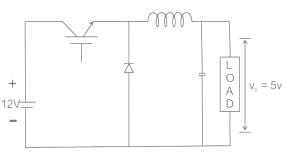Detailed Solution for Test: Steady State Time Domain Analysis of Type-A Chopper - Question 3

For Buck Converter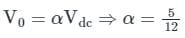The peak to peak ripple current is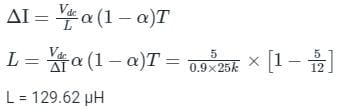*Answer can only contain numeric values
Test: Steady State Time Domain Analysis of Type-A Chopper - Question 4

In chopper circuit the average value of output voltage is controlled by time ratio control (TRC) method. The chopper is operated at a frequency of 5kHz on a 230 V d.c. supply. If the load voltage is 180 V, then the blocking period of thyristor in each cycle is___(in μsec)

Detailed Solution for Test: Steady State Time Domain Analysis of Type-A Chopper - Question 4

Vo = Vdc × Ton × f
f = 5 kHz, Vdc = 230 V, Vo = 180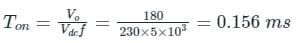but chopping period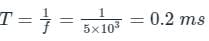blocking period of SCR
Toff = T – Ton = 0.2 – 0.156 = 0.04347 msec = 43.47 μsec

Test: Steady State Time Domain Analysis of Type-A Chopper - Question 5

A dc chopper is fed from constant voltage mains. The duty ratio α of the chopper is progressively increased while the chopper feeds RL load. The per unit current ripple would

Detailed Solution for Test: Steady State Time Domain Analysis of Type-A Chopper - Question 5

Ripple current in the chopper circuit :
Ripple current is the difference between maximum current (Imx) and minimum current (Imin) flowing through the chopper circuit in the steady-state operation of the chopper.
The ripple current of chopper operating in steady-state is given by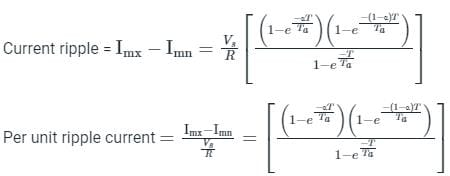Where
α = duty cycle of the chopper, Vs = DC supply voltage, R = load resistance, Ta = time constant = L/R , and T = time period of the chopper
From the above equation, we can observe per unit ripple current is only depends on the time constant (Ta), Time period (T), and duty cycle (α ).
Variation of ripple current with a duty cycle (α) and T/Ta ratio:
We can observe the variation of ripple current with duty cycle and T/Ta ration in the figure is shown below,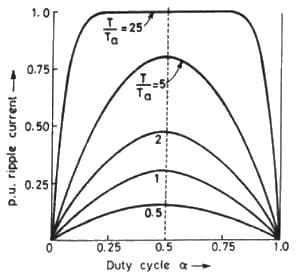• From the above graph, the value of ripple current is increased with an increasing duty cycle (α) up to some instant where the value of α =0.5, and after that, it is decreasing.
• The maximum value of ripple current  is given at the value of α = 0.5
• The maximum value of the ripple current is increased with increasing T/Ta ratio but still, the max ripple current occurs at α = 0.5.

## Power Electronics

5 videos|39 docs|63 tests
 Use Code STAYHOME200 and get INR 200 additional OFF Use Coupon Code
Information about Test: Steady State Time Domain Analysis of Type-A Chopper Page
In this test you can find the Exam questions for Test: Steady State Time Domain Analysis of Type-A Chopper solved & explained in the simplest way possible. Besides giving Questions and answers for Test: Steady State Time Domain Analysis of Type-A Chopper, EduRev gives you an ample number of Online tests for practice

## Power Electronics

5 videos|39 docs|63 tests